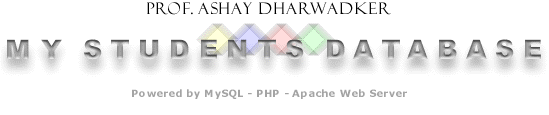| Home | Sign up! | Projects | Seminars | Research Notes | Today's Lecture | About |
 Member ID: 72

## Ravi Purohit

Email
ravipurohit7@hotmail.com

Profile: I am interested in all aspects of mathematics and computer science.

Prof. Ashay Dharwadker's Courses (1):

 Course Semester Grade Add New Course Research Summer 2005 View Update Course

### Seminars (1)

 Seminar ID: 60Course: TopologyTopic: Poincare's Model of Hyperbolic GeometryUpdate Seminar Description: The object of this seminar is to explore Poincare's model of hyperbolic geometry as a perfectly consistent alternative to the usual Euclidean geometry we learn in school (all those theorems about congruent triangles ABC!). If you think about it, one is free to define a "geometry" as any set of consistent axioms about "points", "straight lines" and so on. The important point is that the axioms must be consistent, i.e. one must never be able to prove a statement and its negation from this set of axioms. Also, let us try to select a set of axioms that are not too unrealistic, in the sense that the axioms correspond to the physical behaviour of objects in the real world. For example we wish in some sense that "light travels in a straight line" in our geometry. Imagine a world composed of the interior of a circle C such that the velocity of light at any point inside the circle is equal to the distance of that point from the circumference. Now, it can be proved that rays of light will take the shape of circular arcs with each end perpendicular to the circumference of C. In such a geometry, the properties of "straight lines" defined as light rays will be different from properties of straight lines in usual Euclidean geometry. For example, the axiom of Euclidean geometry that "there is exactly one line parallel to a given line and passing through a point not on the line" will not be true because there will be infinitely many "straight lines" through any point that do not intersect a given "straight line". This is Poincare's model of hyperbolic geometry. We present a simple program using C++ and OpenGL that shows the "straight lines" of this geometry. It was interesting writing the program, since the axioms of the geometry had to be essentially written in C++!### Research Notes (1)

 Research Note ID: 84Course: TopologyTopic: The Riemann SphereUpdate Research Note Description: The complex plane C is not compact. In the study of analytic functions, it is convenient to adjoin a single new point called ¥. This process is called the one-point compactification of C. The resulting compact set C È {¥} is called the Riemann Sphere. How is the topology of the Riemann Sphere defined? For any real number r > 0, D(a, r) denotes the usual disc of radius r centered at a in the complex plane. Let D'(¥, r) be defined as the set of all complex numbers z such that |z| > r. Now define D(¥, r) = D'(¥, r) È {¥} . Then we say that a subset X of the Riemann Sphere is open if and only if X is the union of discs D(a, r) on the Riemann Sphere where a and r are arbitrary. The induced subspace topology on C is the the usual topology of the complex plane. Define the map f : C È {¥} ® R3 by f(reiq) = ((2r cos(q)/(r2+1), (2r sin(q)/(r2+1), (r2-1)/(r2+1)). Then f is a homeomorphism of the Riemann sphere onto the unit sphere in R3. In the picture below, the red rays of the complex plane C are shown mapped onto the corresponding green curves on the Riemann Sphere. The origin of the complex plane is mapped onto the North Pole of the Riemann Sphere and the South Pole corresponds to the adjoined point ¥.Last updated on Thursday, 11th August 2005, 04:02:36 PM.